Courses

# Test: Greedy Techniques- 2

## 20 Questions MCQ Test Algorithms | Test: Greedy Techniques- 2

Description
This mock test of Test: Greedy Techniques- 2 for Computer Science Engineering (CSE) helps you for every Computer Science Engineering (CSE) entrance exam. This contains 20 Multiple Choice Questions for Computer Science Engineering (CSE) Test: Greedy Techniques- 2 (mcq) to study with solutions a complete question bank. The solved questions answers in this Test: Greedy Techniques- 2 quiz give you a good mix of easy questions and tough questions. Computer Science Engineering (CSE) students definitely take this Test: Greedy Techniques- 2 exercise for a better result in the exam. You can find other Test: Greedy Techniques- 2 extra questions, long questions & short questions for Computer Science Engineering (CSE) on EduRev as well by searching above.
QUESTION: 1

### Let G be an undirected connected graph with distinct edge weight. Let emax be the edge with maximum weight and emin the edge with minimum weight. Which of the following statements is false?

Solution:

(a) and (b) are always true. (c) is false because (b) is true. (d) is true because all edge weights are distinct for G.

QUESTION: 2

### Which of the following algorithm can be used to efficiently calculate single source shortest paths in a Directed Acyclic Graph?

Solution:

Using Topological Sort, we can find single source shortest paths in O(V+E) time which is the most efficient algorithm. See following for details. Shortest Path in Directed Acyclic Graph

QUESTION: 3

### Consider the undirected graph below: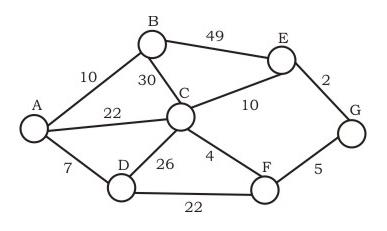Using Prim's algorithm to construct a minimum spanning tree starting with node A, which one of the following sequences of edges represents a possible order in which the edges would be added to construct the minimum spanning tree?

Solution:

A and B are False  : The idea behind Prim’s algorithm is to construct a spanning tree - means all vertices must be connected but here vertices are disconnected C. False. Prim's is a greedy algorithm and At every step, it considers all the edges that connect the two sets, and picks the minimum weight edge from these edges. In this option weight of AB<AD so must be picked up first. D.TRUE. It represents a possible order in which the edges would be added to construct the minimum spanning tree. Prim’s algorithm is also a Greedy algorithm. It starts with an empty spanning tree. The idea is to maintain two sets of vertices. The first set contains the vertices already included in the MST, the other set contains the vertices not yet included. At every step, it considers all the edges that connect the two sets, and picks the minimum weight edge from these edges. After picking the edge, it moves the other endpoint of the edge to the set containing MST.

QUESTION: 4

Consider the following graph: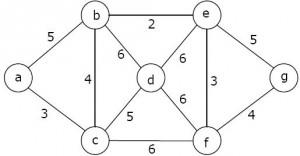Which one of the following is NOT the sequence of edges added to the minimum spanning tree using Kruskal's algorithm?

Solution:

In the sequence (b, e) (e, f) (b, c) (a, c) (f, g) (c, d) given option D, the edge (a, c) of weight 4 comes after (b, c) of weight 3. In Kruskal's Minimum Spanning Tree Algorithm, we first sort all edges, then consider edges in sorted order, so a higher weight edge cannot come before a lower weight edge.

QUESTION: 5

Given a directed graph where weight of every edge is same, we can efficiently find shortest path from a given source to destination using?

Solution:

With BFS, we first find explore vertices at one edge distance, then all vertices at 2 edge distance, and so on.

QUESTION: 6

Consider the weights and values of items listed below. Note that there is only one unit of each item.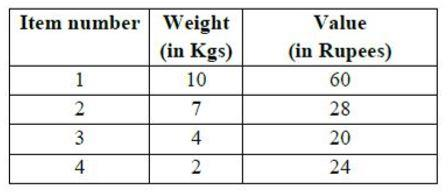The task is to pick a subset of these items such that their total weight is no more than 11 Kgs and their total value is maximized. Moreover, no item may be split. The total value of items picked by an optimal algorithm is denoted by Vopt. A greedy algorithm sorts the items by their value-to-weight ratios in descending order and packs them greedily, starting from the first item in the ordered list. The total value of items picked by the greedy algorithm is denoted by Vgreedy. The value of Vopt − Vgreedy is ______ .

Note -This was Numerical Type question.

Solution: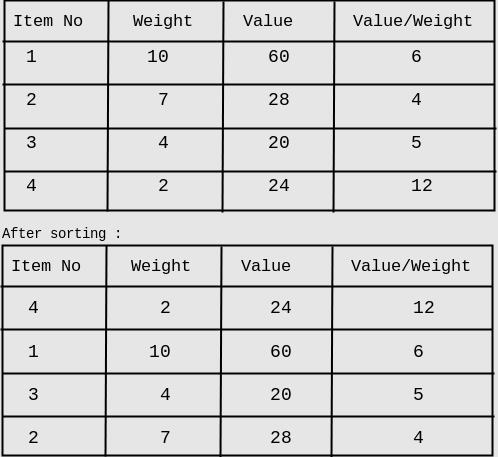First we will pick item_4 (Value weight ratio is highest). Second highest is item_1, but cannot be picked because of its weight. Now item_3 shall be picked. item_2 cannot be included because of its weight. Therefore, overall profit by Vgreedy = 20+24 = 44 Hence, Vopt - Vgreedy = 60-44 = 16 So, answer is 16.

QUESTION: 7

The number of distinct minimum spanning trees for the weighted graph below is ____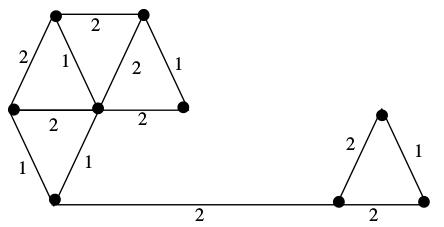Solution:

Below diagram shows a minimum spanning tree. Highlighted (in green) are the edges picked to make the MST.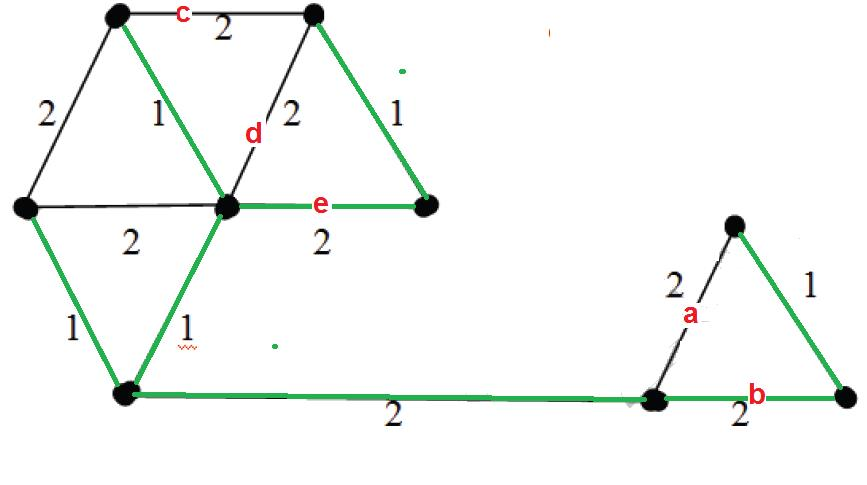In the right side of MST, we could either pick edge ‘a’ or ‘b’. In the left side, we could either pick ‘c’ or ‘d’ or ‘e’ in MST. There are 2 options for one edge to be picked and 3 options for another edge to be picked. Therefore, total 2*3 possible MSTs.

QUESTION: 8

Is the following statement valid about shortest paths? Given a graph, suppose we have calculated shortest path from a source to all other vertices. If we modify the graph such that weights of all edges is becomes double of the original weight, then the shortest path remains same only the total weight of path changes.

Solution:

The shortest path remains same. It is like if we change unit of distance from meter to kilo meter, the shortest paths don't change.

QUESTION: 9

A text is made up of the characters a, b, c, d, e each occurring with the probability 0.11, 0.40, 0.16, 0.09 and 0.24 respectively. The optimal Huffman coding technique will have the average length of:

Solution:

a = 0.11 b = 0.40 c = 0.16 d = 0.09 e = 0.24 we will draw a huffman tree: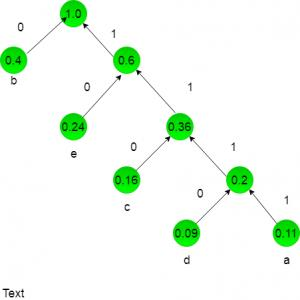now huffman coding for character:

a = 1111
b = 0
c = 110
d = 1111
e = 10
lenghth for each character = no of bits * frequency of occurence:
a = 4 * 0.11
= 0.44
b = 1 * 0.4
=  0.4
c = 3 * 0.16
= 0.48
d = 4 * 0.09
=  0.36
e = 2 * 0.24
= 0.48
Now add these lenght for average length:
0.44 + 0.4 + 0.48 + 0.36 + 0.48 = 2.16

QUESTION: 10

Let s and t be two vertices in a undirected graph G + (V, E) having distinct positive edge weights. Let [X, Y] be a partition of V such that s ∈ X and t ∈ Y. Consider the edge e having the minimum weight amongst all those edges that have one vertex in X and one vertex in Y The edge e must definitely belong to:

Solution:

The minimum weight edge on any s-t cut is always part of MST. This is called Cut Property. This is the idea used in Prim's algorithm. The minimum weight cut edge is always a minimum spanning tree edge. Why B (the weighted shortest path from s to t) is not an answer? See below example, edge 4 (lightest in highlighted red cut from s to t) is not part of shortest path.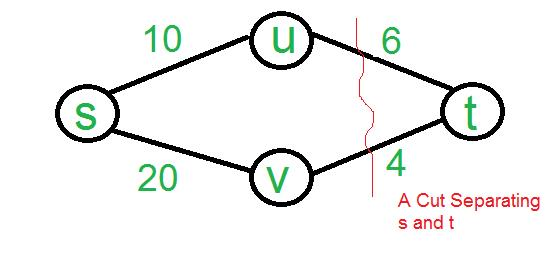QUESTION: 11

Given a weighted graph where weights of all edges are unique (no two edge have same weights), there is always a unique shortest path from a source to destination in such a graph.

Solution:

There can be more than one paths with same weight. Consider a path with one edge of weight 5 and another path with two edges of weights 2 and 3. Both paths have same weights.

QUESTION: 12

Six files F1, F2, F3, F4, F5 and F6 have 100, 200, 50, 80, 120, 150 records respectively. In what order should they be stored so as to optimize act. Assume each file is accessed with the same frequency

Solution:

This question is based on the Optimal Storage on Tape problem which uses greedy approach to find the optimal time to retrieve them.
There are n programs of length L that are to be stored on a computer tape. Associated with each program i is a length Li. So in order to
retrieve these programs most optimally, we need to store them in the non-decreasing order of length Li.
So, the correct order is F3, F4, F1, F5, F6, F2

QUESTION: 13

What is the weight of a minimum spanning tree of the following graph ?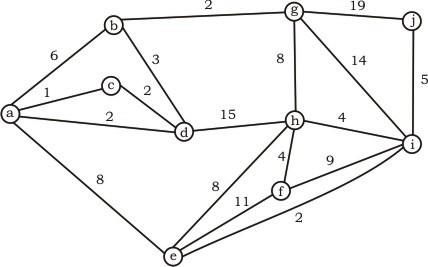Solution:

(a,c), (a,d), (d,b), (b,g), (g,h), (h,f), (h,i), (i,j), (i,e) = 31   Background required - Minimum Spanning Tree (Prims / Kruskal) In these type of questions, always go for kruskal’s algorithm to find out the the minimum spanning tree as it is easy and there are less chances of doing silly mistakes. Algorithm: Always pick the minimum edge weight and try to add to current forest (Collection of Trees) if no cycle is formed else discard. As soon as u have added n-1 edges to the forest, stop and you have got your minimum spanning tree. See the below image for construction of MST of this question.

Weight of minimum spanning tree = Sum of all the edges in Minimum Spanning tree = 31

QUESTION: 14

Which of the following statement(s)is / are correct regarding Bellman-Ford shortest path algorithm?
P: Always finds a negative weighted cycle, if one exist s.
Q: Finds whether any negative weighted cycle is reachable from the source.

Solution:

Bellman-Ford shortest path algorithm is a single source shortest path algorithm. So it can only find cycles which are reachable from a given source, not any negative weight cycle. Consider a disconnected where a negative weight cycle is not reachable from the source at all. If there is a negative weight cycle, then it will appear in shortest path as the negative weight cycle will always form a shorter path when iterated through the cycle again and again.

QUESTION: 15

The number of permutations of the characters in LILAC so that no character appears in its original position, if the two L’s are indistinguishable, is ________ . Note - This question was Numerical Type.

Solution:

There are 3 choices for the first slot, and then 2 for the third slot. That leaves one letter out of I,A,C unchosen and there are 2 slots that one might occupy. After that, the L′s must go in the 2 unfilled slots. Hence the answer is,
3×2×1×2 = 12
Option (A) is correct.

QUESTION: 16

Consider an undirected unweighted graph G. Let a breadth-first traversal of G be done starting from a node r. Let d(r,u) and d(r,v) be the lengths of the shortest paths from r to u and v respectively in G. If u is visited before v during the breadth-first traversal, which of the following statements is correct?

Solution:

d(r, u) and d(r, v) will be equal when u and v are at same level, otherwise d(r, u) will be less than d(r, v)

QUESTION: 17

Let G = (V, E) be an undirected graph with a subgraph G1 = (V1, El). Weights are assigned to edges of G as follows :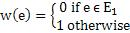A single-source shortest path algorithm is executed on the weighted graph (V, E, w) with an arbitrary vertex ν1 of V1 as the source. Which of the following can always be inferred from the path costs computed?

Solution:

When shortest path shortest path from v1 (one of the vertices in V1) is computed. G1 is connected if the distance from v1 to any other vertex in V1 is greater than 0, otherwise G1 is disconnected.

*Multiple options can be correct
QUESTION: 18

Define Rn to be the maximum amount earned by cutting a rod of length n meters into one or more pieces of integer length and selling them. For i>0, let p[i] denote the selling price of a rod whose length is i meters. Consider the array of prices:
p=1, p=5, p=8, p=9, p=10, p=17, p=18
Which of the following statements is/are correct about R7?

Solution:

According to given data,
Number of pieces    Possible max profits with combination of rod size
7    7 (1, 1, 1, 1, 1, 1, 1)
6    10 (2, 1, 1, 1, 1, 1)
5    13 (2, 2, 1, 1, 1)
4    16 (2, 2, 2, 1)
3    18 (2, 2, 3)
2    18 (6, 1)
1    18 (7)
Therefore, Option (A) and (C) are correct.

QUESTION: 19

The graph shown below 8 edges with distinct integer edge weights. The minimum spanning tree (MST) is of weight 36 and contains the edges: {(A, C), (B, C), (B, E), (E, F), (D, F)}. The edge weights of only those edges which are in the MST are given in the figure shown below. The minimum possible sum of weights of all 8 edges of this graph is ______________.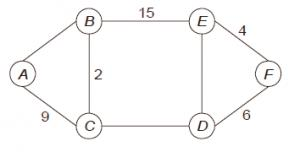Solution:

In every cycle, the weight of an edge that is not part of MST must by greater than or equal to weights of other edges which are part of MST. Since all edge weights are distinct, the weight must be greater. So the minimum possible weight of ED is 7, minimum possible weight of CD is 16 and minimum possible weight of AB is 10. Therefore minimum possible sum of weights is 69.

QUESTION: 20

Let G = (V, E) be a simple undirected graph, and s be a particular vertex in it called the source. For x ∈ V, let d(x) denote the shortest distance in G from s to x. A breadth first search (BFS) is performed starting at s. Let T be the resultant BFS tree. If (u, v) is an edge of G that is not in T, then which one of the following CANNOT be the value of d(u) – d(v)?

Solution:

Note that the given graph is undirected, so an edge (u, v) also means (v, u) is also an edge. Since a shorter path can always be obtained by using edge (u, v) or (v, u), the difference between d(u) and d(v) can not be more than 1.